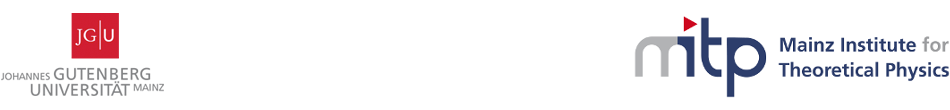#Women at the Intersection of Mathematics and High Energy Physics

6-10 March 2017
Mainz Institute for Theoretical Physics, Johannes Gutenberg University
Europe/Berlin timezone

## Quantum Einstein Equations of Loop Quantum Gravity

8 Mar 2017, 14:00
45m
02.430 (Mainz Institute for Theoretical Physics, Johannes Gutenberg University)

### 02.430

#### Mainz Institute for Theoretical Physics, Johannes Gutenberg University

Staudingerweg 9 / 2nd floor, 55128 Mainz

Kristina Giesel

### Description

In this talk we give a brief review on the conceptual and mathematical framework underlying loop quantum gravity with a focus on its dynamics encoded in the so called quantum Einstein equations. We will discuss how the notion of Dirac observables in the context of general relativity can be used to derive a reduced phase space quantization for loop quantum gravity. Furthermore, we explain how the quantum Einstein equations can be formulated in this context and discuss some recent progress and results.

### Summary

In this talk we give a brief review on the conceptual and mathematical framework underlying loop quantum gravity with a focus on its dynamics encoded in the so called quantum Einstein equations. We will discuss how the notion of Dirac observables in the context of general relativity can be used to derive a reduced phase space quantization for loop quantum gravity. Furthermore, we explain how the quantum Einstein equations can be formulated in this context and discuss some recent progress and results.

### Presentation Materials

There are no materials yet.